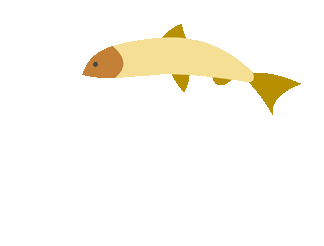top of page## Solving Equations Games

##### Solving Equations (Resources)
1. Solving Equations

2. Solving One-Step Equations
Good notes, and examples on this site.

3. Cool Math
Good Step by step directions for solving equations with fractions and decimals.

##### Solving Equations (Games)
1. Soccer Equations
Solve the equations correctly and take your best shot at the keeper!

2. Hoop Shoot
Solve equations with all four operations and then shoot some hoops.

3. IXL Math Practice
Solve these single step equations with whole numbers!bottom of page